### MEMO 2012 pojedinačno problem 4

Kvaliteta:
Avg: 4,0
Težina:
Avg: 6,0
The sequence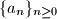$\{ a_n \} _ { n \ge 0 }$ is defined by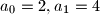$a_0 = 2 , a_1 = 4$ and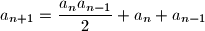for all positive integers$n$. Determine all prime numbers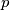$p$ for which there exists a positive integer$m$ such that$p$ divides the number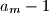$a_m - 1$.
Izvor: Srednjoeuropska matematička olimpijada 2012, pojedinačno natjecanje, problem 4